# TMac: Tensor completion by parallel Matrix factorization

Yangyang Xu, Ruru Hao, Wotao Yin, and Zhixun Su

## Background

Higher-order low-rank tensors with missing values naturally arise in many applications including hyperspectral data recovery, video inpainting, seismic data reconstruction, and so on. These problems can be formulated as low-rank tensor completion (LRTC). Existing methods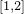for LRTC employ matrix nuclear-norm minimization and use the singular value decomposition (SVD) in their algorithms, which become very slow or even not applicable for large-scale problems. To tackle this difficulty, we apply low-rank matrix factorization to each mode unfolding of the tensor in order to enforce low-rankness and update the matrix factors alternatively, which is computationally much cheaper than SVD.

## Our method

We aim at recovering a low-rank tensor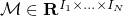from partial observations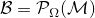, whereis the index set of observed entries, and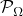keeps the entries inand zeros out others. We apply low-rank matrix factorization to each mode unfolding ofby finding matrices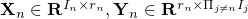such that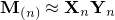for, where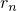is the estimated rank, either fixed or adaptively updated. Introducing one common variableto relate these matrix factorizations, we solve the following model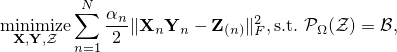whereand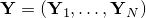. In the model,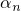,, are weights and satisfy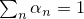. We use alternating least squares method to solve the model.

## Results

Our model is non-convex jointly with respect to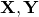and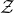. Although a global solution is not guaranteed, we demonstrate by numerical experiments that our algorithm can reliably recover a wide variety of low-rank tensors, such as the following phase transition plots. In the picture, each target tensor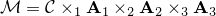, where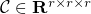and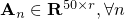have Gaussian random entries. (a) FaLRTC: the tensor completion method in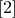. (b) MatComp: first reshape the tensor as a matrix and then use the matrix completion solver LMaFit in. (c) TMac-fix: our method withandfixed to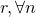. (d) TMac-inc: our method withand using rank-increasing strategy starting from. (e) TMac-dec: our method withand using rank-decreasing strategy starting from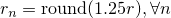.

The results show that our method performs much better than the other two methods.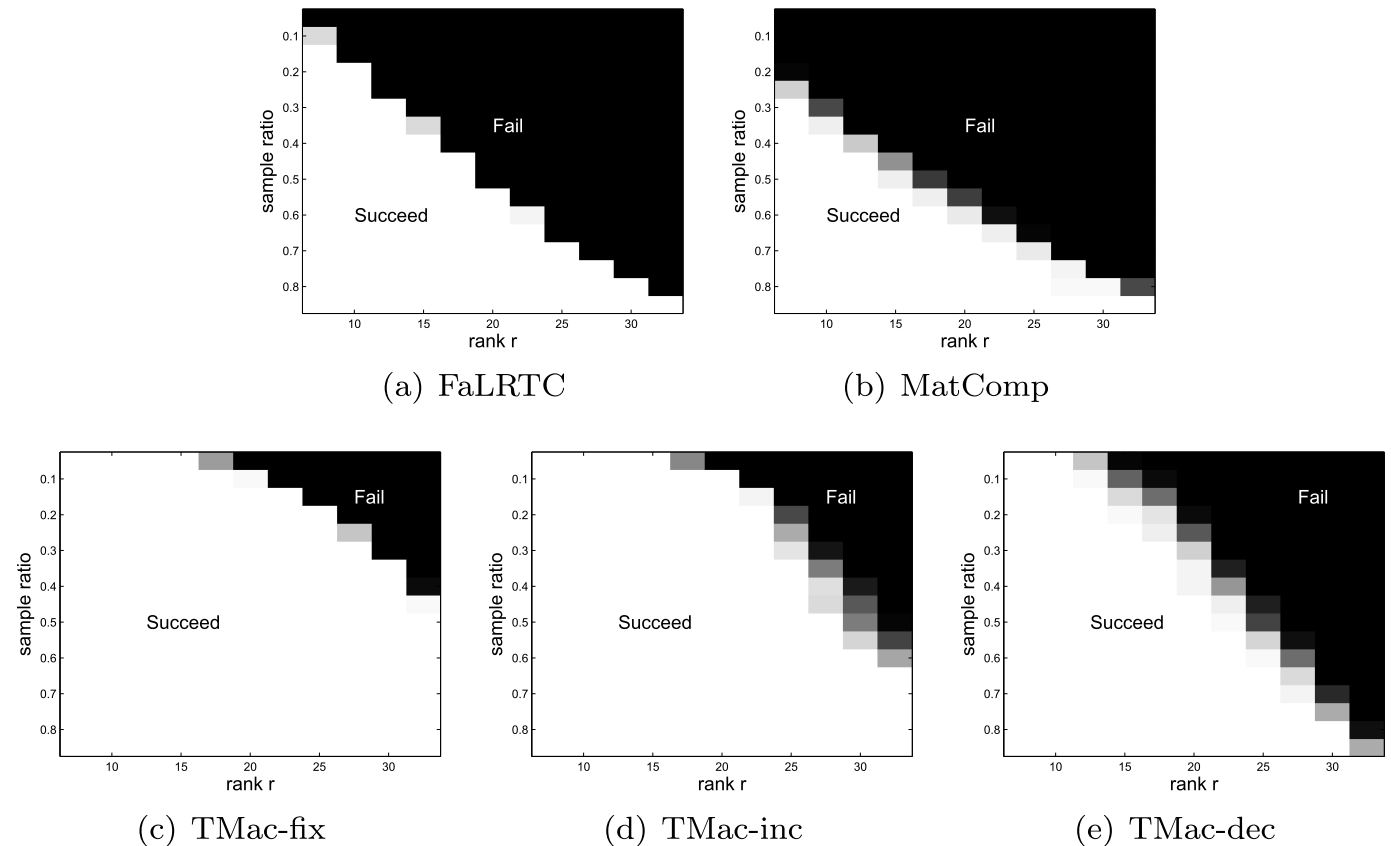## Matlab code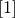S. Gandy, B. Recht, and I. Yamada, Tensor completion and low-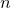-rank tensor recovery via convex optimization, Inverse Problems, 27(2011), p. 025010.J. Liu, P. Musialski, P. Wonka, and Jieping Ye, Tensor completion for estimating missing values in visual data, IEEE Transactions on Pattern Analysis and Machine Intelligence, (2013), pp. 208-220.Z. Wen, W. Yin, and Y. Zhang, Solving a low-rank factorization model for matrix completion by a nonlinear successive over-relaxation algorithm, Mathematical Programming Computation, (2012), pp. 1-29